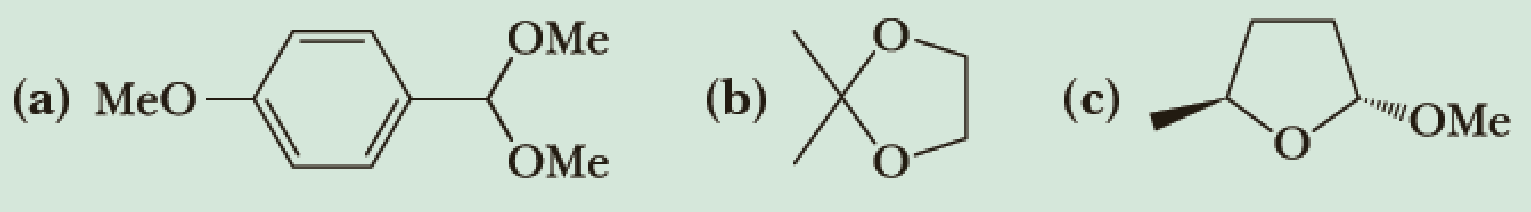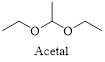# Hydrolysis of an acetal in aqueous acid gives an aldehyde or a ketone and two molecules of alcohol or one molecule of a diol. Draw the structural formulas for the products of hydrolysis of the following acetals in aqueous acid.### Organic Chemistry

8th Edition
William H. Brown + 3 others
Publisher: Cengage Learning
ISBN: 9781305580350

#### Solutions

Chapter
Section### Organic Chemistry

8th Edition
William H. Brown + 3 others
Publisher: Cengage Learning
ISBN: 9781305580350
Chapter 16.7, Problem 16.6P
Textbook Problem
83 views

## Hydrolysis of an acetal in aqueous acid gives an aldehyde or a ketone and two molecules of alcohol or one molecule of a diol. Draw the structural formulas for the products of hydrolysis of the following acetals in aqueous acid.(a)

Interpretation Introduction

Interpretation:

The structural formulas for the products of hydrolysis of the given acetal in aqueous acid has to be drawn.

Concept introduction:

Acetals are a group of organic compound that contain two alkoxy (-OR) groups on the same carbon atom.Acetals can be hydrolysed by addition of dilute acid. The excess of water drives the equilibrium toward the formation of the ketone or aldehyde and two molecules of alcohol or one molecule of diol.

### Explanation of Solution

Hydrolysis of an acetal in aqueous acid gives an aldehyde or ketone and two molecules of alcohol or a molecule of a diol.

The hydrolysis of given acetal in aqueous acid can be shown as follows,

(b)

Interpretation Introduction

Interpretation:

The structural formulas for the products of hydrolysis of the given acetal in aqueous acid has to be drawn.

Concept introduction:

Acetals are a group of organic compound that contain two alkoxy (-OR) groups on the same carbon atom.Acetals can be hydrolysed by addition of dilute acid. The excess of water drives the equilibrium toward the formation of the ketone or aldehyde and two molecules of alcohol or one molecule of diol.

(c)

Interpretation Introduction

Interpretation:

The structural formulas for the products of hydrolysis of the given acetal in aqueous acid has to be drawn.

Concept introduction:

Acetals are a group of organic compound that contain two alkoxy (-OR) groups on the same carbon atom.Acetals can be hydrolysed by addition of dilute acid. The excess of water drives the equilibrium toward the formation of the ketone or aldehyde and two molecules of alcohol or one molecule of diol.

### Still sussing out bartleby?

Check out a sample textbook solution.

See a sample solution

#### The Solution to Your Study Problems

Bartleby provides explanations to thousands of textbook problems written by our experts, many with advanced degrees!

Get Started